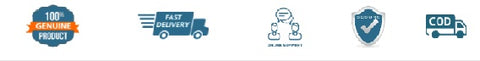# NCERT Mathematics Exemplar Problem for Class 11 - latest edition as per NCERT/CBSE

SKU:3MNCERT312
Price : Rs. 176.00
Sale price Rs. 176.00 Price : Rs. 192.00
Sold out
Unit price
per
Shipping calculated at checkout.

1. Unit 1(Sets)
2. Unit 2(Relations And Functions)
3. Unit 3(Trigonometric Functions)
4. Unit 4(Principle Of Mathematical Induction)
5. Unit 5(Complex Numbers And Quadratic Equations)
6. Unit 6(Linear Inequalities)
7. Unit 7(Permutations And Combinations)
8. Unit 8(Binomial Theorem)&bull; Unit 9(Sequence And Series)
9. Unit 10(Straight Lines)
10. Unit 11(Conic Sections)
11. Unit 12(Introduction To Three Dimensional Geometry)
12. Unit 13(Limits And Derivatives)
13. Unit 14(Mathematical Reasoning)
14. Unit 15(Statistics)
15. Unit 16(Probability)

(All books are 100℅ original and as per the latest NCERT syllabus. Prices includes Rs. 32 per book mandatory binding charges.)